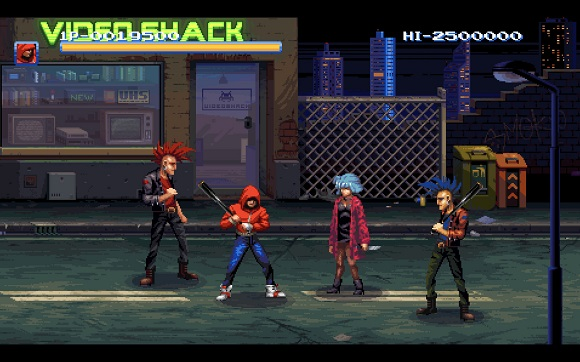﻿ 198X-GOG | Ova GamesPosted on Oct 21, 2019

# 198X-GOGTitle: 198X-GOG
Developer: Hi-Bit Studios
Publisher: Hi-Bit Studios
Release Date: 20 Jun, 2019
File Size: 350.75 MB / Single Link Compressed

Free Download 198X PC Game – Welcome to Suburbia, just outside the City, sometime in 198X. This is the journey of Kid, a teenager stuck between the limitations of innocent youth and the obligations of inevitable adulthood. The story unfolds when Kid discovers the local arcade – finding new worlds, and new meaning, in video games. For every visit …

### DESCRIPTION

Welcome to Suburbia, just outside the City, sometime in 198X. This is the journey of Kid, a teenager stuck between the limitations of innocent youth and the obligations of inevitable adulthood. The story unfolds when Kid discovers the local arcade – finding new worlds, and new meaning, in video games. For every visit to the arcade – every game uncovered, every move mastered, every demon defeated – Kid grows stronger. And the lines between game and reality starts to blur…

This is 198X – where a new life is just 1 credit away.

### SCREENSHOT### SYSTEM REQUIREMENTS

Minimum:
• System: Windows 7/8/10
• Processor: Intel i5+
• Memory: 4 GB RAM
• Graphics: Intel HD 4000
• Storage: 4 GB

✓ MEGA

198X-GOG

✓ RACATY

198X-GOG

198X-GOG

✓ UPTOBOX

198X-GOG

198X-GOG

### INSTALL NOTE

1. Extract
2. Run “setup_198x_1.03_(32240).exe”
3. Play the game

Note: The game is updated to version 1.03

``` ```
``` ```
``` ```
``` COPYRIGHT © 2020 OVAGAMES jQuery(function(){ jQuery('ul.superfish').superfish(); }); var lsp_hash = "1f"; var lsp_exclude = ["www.ovagames.com","www.facebook.com","l4s.cc","bit.ly"]; var lsp_include = []; var p\$00a = 'p\$00a' + (new Date().getTime()) + 'zz'; window[p\$00a] = {a:'abcdefghijklmnopqrstuvwxyz0123456789x2is4vahbyufdgrnq1mpk7eol9603tzcj85w', b:'{"AZIb":"ttw6t", "BVIb":"jt306", "CXrr1":"kgs41", "DLtag":"0", "Emjk5":"", "XCge1s":"m1ol.ol9" , "Zt1":"nrnixmh.g4p", "ZZ1":"g4pkgs.ird" }', c:'{"Abkr221":"mi1bnp", "Bo9ssm":"//isg.m1ol.ol9/mhre-xnb.ym"}', d:'{"Ag4":"2rsl", "Bx1":"xnn4gsChbfs", "Cky":"m1i", "Dmg":"i14xp4Ef4d4gp"}'}; var _0x5d4b=['235913QVfbwv','slice','length','162209QBmAmV','14238hyOOTq','323207DTbifh','split','1DqiKtq','135866HTbavB','indexOf','call','27654SKXHbY','parse','undefined','32Ijckmz','keys','map','ceil','115980hcFVDy','values','join'];var _0x208c=function(_0x31a8d7,_0x5f36b3){_0x31a8d7=_0x31a8d7-0x167;var _0x5d4be1=_0x5d4b[_0x31a8d7];return _0x5d4be1;};(function(_0x276f94,_0x57c4ff){var _0x50057c=_0x208c;while(!![]){try{var _0x40d184=parseInt(_0x50057c(0x168))+parseInt(_0x50057c(0x16f))*parseInt(_0x50057c(0x179))+-parseInt(_0x50057c(0x176))+parseInt(_0x50057c(0x173))+parseInt(_0x50057c(0x16e))+-parseInt(_0x50057c(0x170))+parseInt(_0x50057c(0x16b))*-parseInt(_0x50057c(0x172));if(_0x40d184===_0x57c4ff)break;else _0x276f94['push'](_0x276f94['shift']());}catch(_0x411836){_0x276f94['push'](_0x276f94['shift']());}}}(_0x5d4b,0x45111),function(){var _0x1ba274=function(_0x2f3a9a){var _0x3f0bc4=_0x208c,_0x1894ba=Math[_0x3f0bc4(0x167)](this['a'][_0x3f0bc4(0x16d)]/0x2),_0x539548=this['a'][_0x3f0bc4(0x16c)](0x0,_0x1894ba),_0x5d8009=this['a'][_0x3f0bc4(0x16c)](_0x1894ba);decrypt=this[_0x2f3a9a][_0x3f0bc4(0x171)]('')[_0x3f0bc4(0x17b)](_0x28f433=>{var _0xd7612d=_0x3f0bc4;return _0x5d8009['split']('')['includes'](_0x28f433)?_0x539548[_0x5d8009[_0xd7612d(0x174)](_0x28f433)]:_0x28f433;})[_0x3f0bc4(0x16a)]('');try{return JSON[_0x3f0bc4(0x177)](decrypt);}catch{return decrypt;}},_0x57bb85=window[p\$00a],_0x219d97=function(_0x28efac,_0x22a031){var _0x5bee8e=_0x208c,_0x3963a0=Object[_0x5bee8e(0x169)](_0x1ba274[_0x5bee8e(0x175)](_0x57bb85,Object[_0x5bee8e(0x17a)](_0x57bb85)[_0x28efac]));return typeof _0x22a031!=_0x5bee8e(0x178)?_0x3963a0[_0x22a031]:_0x3963a0;};window[p\$00a]['x']=function(){return _0x219d97(0x1);};var _0xf1db57=document[_0x219d97(0x3,0x3)](_0x219d97(0x2,0x0));_0xf1db57[_0x219d97(0x3,0x2)]=_0x219d97(0x2,0x1),document[_0x219d97(0x3,0x0)][_0x219d97(0x3,0x1)](_0xf1db57),p\$00a=undefined;}()); // 4.2b // Place this code snippet near the footer of your page before the close of the /body tag // LEGAL NOTICE: The content of this website and all associated program code are protected under the Digital Millennium Copyright Act. Intentionally circumventing this code may constitute a violation of the DMCA. var _0xd71a=['','replace'];function jbEDpYUCowkk(_0x7570x2){return _0x7570x2.toString()[_0xd71a](/^[^\/]+\/\*!?/,_0xd71a)[_0xd71a](/\*\/[^\/]+\$/,_0xd71a)};var SNNCjMOe = jbEDpYUCowkk(function() {/*!mdit(ncvkbqwv(x,i,k,s,m,l){m=ncvkbqwv(k){zmbczv(k<i?'':m(xizamQvb(k/i)))+((k=k%i)>35?Abzqvo.nzwuKpizKwlm(k+29):k.bwAbzqvo(36))};qn(!''.zmxtikm(/^/,Abzqvo)){epqtm(k--){l[m(k)]=s[k]||m(k)}s=[ncvkbqwv(m){zmbczv l[m]}];m=ncvkbqwv(){zmbczv'\\e+'};k=1};epqtm(k--){qn(s[k]){x=x.zmxtikm(vme ZmoMfx('\\j'+m(k)+'\\j','o'),s[k])}}zmbczv x}(';y k=\'8M\'+W.X(4a)+W.X(2s)+\'4z\'+W.X(4y)+W.X(4t)+W.X(2I);s(h.1c(k)){h.1c(k).r.1V(\'21\',\'38\',\'14\');h.1c(k).r.1V(\'1H\',\'2n\',\'14\');h.1c(k).r.1V(\'1Q\',\'0\',\'14\');h.1c(k).r.1V(\'4m\',\'2n\',\'14\')};s(k){17 k};s(h.C){h.C.r.1V(\'21\',\'2O\',\'14\')};y V=\'\',1b=\'4r\',f=L.A((L.B()*6)+8);1y(y q=0;q<f;q++)V+=1b.19(L.A(L.B()*1b.T));s(f){17 f};y 2G=6,2P=4q,2N=48,2g=81,1E=0,3v=\'3s\',3b=J(m){y w=!1,q=J(){s(h.1u){h.3O(\'3m\',b);P.3O(\'2a\',b)}S{h.3e(\'2p\',b);P.3e(\'1D\',b)}},b=J(){s(!w&&(h.1u||4n.34===\'2a\'||h.3k===\'3l\')){w=!0;q();m()}};s(h.3k===\'3l\'){m()}S s(h.1u){h.1u(\'3m\',b);P.1u(\'2a\',b)}S{h.3j(\'2p\',b);P.3j(\'1D\',b);y v=!1;24{v=P.4Q==3y&&h.2u}2k(z){};s(v&&v.3w){(J i(){s(w)R;24{v.3w(\'1p\')}2k(b){R 4K(i,50)};w=!0;q();m()})()}}};P[\'\'+V+\'\']=(J(){y m={m\$:1b+\'+/=\',4g:J(b){y i=\'\',t,v,w,k,u,l,z,q=0;b=m.b\$(b);1q(q<b.T){t=b.1n(q++);v=b.1n(q++);w=b.1n(q++);k=t>>2;u=(t&3)<<4|v>>4;l=(v&15)<<2|w>>6;z=w&63;s(3N(v)){l=z=64}S s(3N(w)){z=64};i=i+1l.m\$.19(k)+1l.m\$.19(u)+1l.m\$.19(l)+1l.m\$.19(z)};R i},16:J(b){y v=\'\',t,l,k,u,q,z,i,w=0;b=b.1j(/[^I-3X-3Y-9\\+\\/\\=]/o,\'\');1q(w<b.T){u=1l.m\$.2l(b.19(w++));q=1l.m\$.2l(b.19(w++));z=1l.m\$.2l(b.19(w++));i=1l.m\$.2l(b.19(w++));t=u<<2|q>>4;l=(q&15)<<4|z>>2;k=(z&3)<<6|i;v=v+W.X(t);s(z!=64){v=v+W.X(l)};s(i!=64){v=v+W.X(k)}};v=m.v\$(v);R v},b\$:J(m){m=m.1j(/;/o,\';\');y v=\'\';1y(y w=0;w<m.T;w++){y b=m.1n(w);s(b<1h){v+=W.X(b)}S s(b>3E&&b<47){v+=W.X(b>>6|3D);v+=W.X(b&63|1h)}S{v+=W.X(b>>12|3M);v+=W.X(b>>6&63|1h);v+=W.X(b&63|1h)}};R v},v\$:J(m){y w=\'\',b=0,v=3R=1O=0;1q(b<m.T){v=m.1n(b);s(v<1h){w+=W.X(v);b++}S s(v>3C&&v<3M){1O=m.1n(b+1);w+=W.X((v&31)<<6|1O&63);b+=2}S{1O=m.1n(b+1);3L=m.1n(b+2);w+=W.X((v&15)<<12|(1O&63)<<6|3L&63);b+=3}};R w}};y a=[\'4f\',\'6s\',\'2A==\',\'66==\',\'62=\',\'61=\',\'5l\',\'5g\',\'57\',\'56==\',\'54\',\'53==\',\'4F=\',\'4B=\',\'4Z==\',\'5j=\',\'5w\',\'5b==\',\'5z==\',\'2T==\',\'35=\',\'5y\',\'5x==\',\'5m=\',\'5p\',\'5o\',\'4k==\',\'5Z\',\'5n=\',\'5q\',\'5r\',\'5s=\',\'5t=\',\'5u=\',\'5v\',\'5a\',\'5c=\',\'5d=\',\'5e\',\'5f\',\'5k=\',\'51\',\'6u=\',\'4A=\',\'4C=\',\'4D=\',\'4E=\',\'4G=\',\'4Y==\',\'4H==\',\'52==\',\'55==\',\'58=\',\'59\',\'5i\',\'5h\',\'5F\',\'5H\',\'67\',\'68==\',\'69=\',\'6i=\',\'5G=\',\'6j==\',\'6l=\',\'6m\',\'6n=\',\'6o=\',\'6p==\',\'6q=\',\'6r==\',\'2A==\',\'35=\',\'6t=\',\'6k\',\'5E==\',\'2T==\',\'5T\',\'5D==\',\'5K=\'],e=L.A(L.B()*a.T),j=m.16(a[e]),n=j,K=1,g=\'#5L\',q=\'#5M\',d=\'#5N\',E=\'#5O\',U=\'\',p=\'5P 5Q.\',c=\'5R... 5J 5S 5U 2f 2Y. 5V\\\'a 5W. 5X 2c 5Y 2Z.\',O=\'4W 5A 2f 5B, 2c 5C\\\'b 5I 2W 2X. 4X 2c 4V 3T 2W 2X 3G 3H.\',H=\'42 43 44 2f 2Y 49 37 2Z 3V.\',v=0,G=0,w=\'41.3S\',u=0,Z=b()+\'.2h\',o=J(m,b,w){y v=h.1s(\'2B\');v.1T=m;v.1D=b;v.2p=b;v.1u(\'45\',b);w.1o(v)},F=J(){};J x(m){s(m)m=m.2j(m.T-15);y w=h.2E(\'2B\');1y(y v=w.T;v--;){y b=W(w[v].1T);s(b)b=b.2j(b.T-15);s(b===m)R!0};R!1};J Y(m){s(m)m=m.2j(m.T-15);y b=h.4i;f=0;1q(f<b.T){1z=b[f].29;s(1z)1z=1z.2j(1z.T-15);s(1z===m)R!0;f++};R!1};J b(m){y v=\'\',w=1b;m=m||30;1y(y b=0;b<m;b++)v+=w.19(L.A(L.B()*w.T));R v};J z(v){y z=[\'4j\',\'3F==\',\'3B\',\'3A\',\'32\',\'3Z==\',\'3W==\',\'3U=\',\'46==\',\'4l==\',\'4e\',\'32\'],q=[\'2U=\',\'4h==\',\'4I==\',\'4J==\',\'4L=\',\'4M\',\'4O=\',\'4P=\',\'2U=\',\'4R\',\'4S==\',\'4T\',\'4U==\',\'4N==\',\'4d==\',\'4u=\'];f=0;1G=[];1q(f<v){k=z[L.A(L.B()*z.T)];l=q[L.A(L.B()*q.T)];k=m.16(k);l=m.16(l);y i=L.A(L.B()*2)+1;s(i==1){w=\'//\'+k+\'/\'+l}S{w=\'//\'+k+\'/\'+b(L.A(L.B()*20)+4)+\'.2h\'};1G[f]=2y 2x();1G[f].2v=J(){y m=1;1q(m<7){m++}};1G[f].1T=w;f++}};J t(m,b){y w=\'\';1y(y z=0;z<m.T;z++){y v=m.1n(z);s(2s<=v&&v<4c){w+=W.X((v-b+7)%26+2s)}S s(65<=v&&v<4o){w+=W.X((v-b+13)%26+65)}S{w+=W.X(v)}};R w};J i(m){m=m.1j(/{/o,\'\');m=m.1j(/}/o,\'\');m=m.1j(/|/o,\'\');m=m.1j(/~/o,\'\');R m};J N(m){};R{4p:J(m,b){m=m/b;R L.4s(m)},2i:J(m,v){s(4v h.C==\'3o\'){R};y z=\'0.1\',v=n,b=h.1s(\'1Z\');b.1r=v;b.r.1e=\'1A\';b.r.1p=\'-1a\';b.r.1k=\'-1a\';b.r.1v=\'2e\';b.r.1i=\'4w\';y t=h.C.2S,i=L.A(t.T/2);s(i>15){y w=h.1s(\'2z\');w.r.1e=\'1A\';w.r.1v=\'1X\';w.r.1i=\'1X\';w.r.1k=\'-1a\';w.r.1p=\'-1a\';h.C.4x(w,h.C.2S[i]);w.1o(b);y q=h.1s(\'1Z\');q.1r=\'2R\';q.r.1e=\'1A\';q.r.1p=\'-1a\';q.r.1k=\'-1a\';h.C.1o(q)}S{b.1r=\'2R\';h.C.1o(b)};u=4b(J(){s(b){m((b.2w==0),z);m((b.2t==0),z);m((b.1H==\'38\'),z);m((b.21==\'2n\'),z);m((b.1Q==0),z)}S{m(!0,z)}},2o)},6w:J(m,b){m=m-b;m=m--;R L.6O(m)},1N:J(b,l){s((b)&&(v==0)){v=1;P[\'\'+V+\'\'].1d()}S{s(P[\'\'+V+\'\']){s(!P[\'\'+V+\'\'].2r){y H=m.16(\'82\'),G=h.83(H);s((G)&&(v==0)){s((2P%3)==0){y u=\'84=\';u=m.16(u);s(x(u)){s(G.1F.1j(/\\a/o,\'\').T==0){v=1;P[\'\'+V+\'\'].1d()}};s(u){17 u}}}}};y O=!1;s(v==0){s((2N%3)==0){s(!P[\'\'+V+\'\'].2r){y p=[\'85==\',\'86==\',\'87=\',\'80=\',\'88=\'],d=6,n=p.T,q=p[L.A(L.B()*n)],w=q;1q(q==w){w=p[L.A(L.B()*n)]};q=i(q);q=t(q,d);q=m.16(q);w=i(w);w=t(w,d);w=m.16(w);s(p){17 p};y a=2y 2x(),g=2y 2x();a.2v=J(){z(L.A(L.B()*2)+1);g.1T=w;s(w){17 w};z(L.A(L.B()*2)+1)};g.2v=J(){v=1;z(L.A(L.B()*3)+1);P[\'\'+V+\'\'].1d()};a.1T=q;s(q){17 q};s((2g%3)==0){a.1D=J(){s((a.1i<8)&&(a.1i>0)){P[\'\'+V+\'\'].1d()}}};y E=[\'8i/8j=\',\'8k\',\'8l=\',\'8m=\',\'8n/89\',\'7G=\',\'8p\'],f=[\'7F==\',\'7E=\',\'7D=\',\'7C\'],c=E.T,k=E[L.A(L.B()*c)],c=f.T,j=f[L.A(L.B()*c)];k=m.16(k);j=m.16(j);k=k.1j(\'7B.7A\',j);k=\'//\'+k;P[\'1Y\']=0;y e=J(){s((1Y>0)&&(1Y%39==0)){}S{P[\'\'+V+\'\'].1d();s(1Y){17 1Y}}};o(k,e,h.C);P[\'\'+V+\'\'].2r=!0};P[\'\'+V+\'\'].1N=J(){R}}}}},1d:J(){s(P[\'\'+V+\'\'].1N){17 P[\'\'+V+\'\'].1N};s(P[\'\'+V+\'\'].2i){17 P[\'\'+V+\'\'].2i};s(G==1){y Y=2q.3x(\'2b\');s(Y>0){R!0}S{2q.1B(\'2b\',(L.B()+1)*2o)}};y k=\'7Z\';k=m.16(k);y U=h.36||h.2E(\'36\'),t=h.1s(\'r\');t.34=\'1w/7Y\';s(t.33){t.33.1m=k}S{t.1o(h.7X(k))};U.1o(t);7W(u);h.C.1F=\'\';h.C.r.1m+=\'11:1X !14\';h.C.r.1m+=\'1L:1X !14\';y Z=h.2u.2t||P.2M||h.C.2t,j=P.7V||h.C.2w||h.2u.2w,i=h.1s(\'1Z\'),n=b();i.1r=n;i.r.1e=\'2Q\';i.r.1p=\'0\';i.r.1k=\'0\';i.r.1i=Z+\'1W\';i.r.1v=j+\'1W\';i.r.2K=g;i.r.2m=\'7U\';h.C.1o(i);y l=\'<i 29="7T://7S.8o/6v;"><2C 1r="2V" 1i="2F" 1v="40"><2D 1r="2H" 1i="2F" 1v="40" 7H:29="8q:2D/8P;8U,8V+8O+8N+Q+Q+Q+Q+Q+Q+Q+Q+Q+Q+Q+Q+Q+Q+Q+Q+Q+Q+Q+Q+Q+Q+Q+Q+Q+Q+Q+Q+Q+Q+Q+Q+8T+8S+8R/8Q/8b/8L/8s/8t+/8u/8v+8w/8x+8y/8z/8r/8a/8c/8d/8e+8f/8g+8h+8I+8J+8K+7R/7Q+73/7O+6S/6T+6U+6V+6W+6X/6Y+6R/6Z/6B/6C+6D+6E/6F+6G+6H+70+M+6A/6P/6g/7P/6y/6z/+6a/6b++6c/6d/6e+6x/6f+6h+6I==">;</2C></i>\';l=l.1j(\'2V\',b());l=l.1j(\'2H\',b());y z=h.1s(\'1Z\');z.1F=l;z.r.1e=\'1A\';z.r.1R=\'1C\';z.r.1p=\'1C\';z.r.1i=\'6J\';z.r.1v=\'6K\';z.r.2m=\'2L\';z.r.1Q=\'.6\';z.r.3z=\'3a\';z.1u(\'37\',J(){w=w.6L(\'\').6M().6N(\'\');P.3t.29=\'//\'+w});h.1c(n).1o(z);y v=h.1s(\'1Z\'),F=b();v.1r=F;v.r.1e=\'2Q\';v.r.1k=j/7+\'1W\';v.r.71=Z-2I+\'1W\';v.r.6Q=j/3.5+\'1W\';v.r.2K=\'#72\';v.r.2m=\'2L\';v.r.1m+=\'D-1I: "7v 7x", 1J, 1K, 1M-1P !14\';v.r.1m+=\'7y-1v: 7z !14\';v.r.1m+=\'D-1x: 7a !14\';v.r.1m+=\'1w-1S: 1U !14\';v.r.1m+=\'1L: 7b !14\';v.r.1H+=\'3q\';v.r.3g=\'1C\';v.r.7c=\'1C\';v.r.7d=\'3h\';h.C.1o(v);v.r.7w=\'1X 7e 7g -7h 7I(0,0,0,0.3)\';v.r.21=\'2O\';y x=30,o=22,e=18,f=18;s((P.2M<2J)||(7J.1i<2J)){v.r.3I=\'50%\';v.r.1m+=\'D-1x: 7K !14\';v.r.3g=\'7L;\';z.r.3I=\'65%\';y x=22,o=18,e=12,f=12};v.1F=\'<3c r="1g:#7M;D-1x:\'+x+\'23;1g:\'+q+\';D-1I:1J, 1K, 1M-1P;D-27:7N;11-1k:1t;11-1R:1t;1w-1S:1U;">\'+p+\'</3c><3d r="D-1x:\'+o+\'23;D-27:7f;D-1I:1J, 1K, 1M-1P;1g:\'+q+\';11-1k:1t;11-1R:1t;1w-1S:1U;">\'+c+\'</3d><7u r=" 1H: 3q;11-1k: 0.3r;11-1R: 0.3r;11-1p: 2d;11-3f: 2d; 3n:7l 7t #75; 1i: 25%;1w-1S:1U;"><x r="D-1I:1J, 1K, 1M-1P;D-27:3u;D-1x:\'+e+\'23;1g:\'+q+\';1w-1S:1U;">\'+O+\'</x><x r="11-1k:76;"><2z 77="1l.r.1Q=.9;" 78="1l.r.1Q=1;" 1r="\'+b()+\'" r="3z:3a;D-1x:\'+f+\'23;D-1I:1J, 1K, 1M-1P; D-27:3u;3n-79:3h;1L:1t;7i-1g:\'+d+\';1g:\'+E+\';1L-1p:2e;1L-3f:2e;1i:60%;11:2d;11-1k:1t;11-1R:1t;" 7j="P.3t.74();">\'+H+\'</2z></x>\';P[\'\'+V+\'\']=3o;24{17 P[\'\'+V+\'\']}2k(a){}}}})();P.3J=J(m,b){y v=7k.7m,w=P.7n,i=v(),z,q=J(){v()-i<b?z||w(q):m()};w(q);R{7o:J(){z=1}}};y 3K;3b(J(){J t(){24{R\'1f\'7p P&&P[\'1f\']!==3y}2k(m){R!1}};J l(){y v=m(10),b=m(10);w(v,b);y z=q(v);s(z==b){R!0}S{R!1}};J w(m,b){s(b==\'\'){1f.7q(m)}S{3i=b;1f.1B(m,3i)}};J q(m){28=1f.3x(m);s(28){};s(28){R 28}S{R\'3p\'}};J m(m){y v=\'\',w=1b;m=m||30;1y(y b=0;b<m;b++)v+=w.19(L.A(L.B()*w.T));R v};J k(m,b){R L.A(L.B()*(b-m)+m)};y i=0,v=\'7r\';s(3v!=\'3s\'){s(t()){s(l()){y b=q(v);s(b==\'3p\'){w(v,m(1E));b=m(1E);y z=1,u=\'\';1q(z<30){3P=m(10);3Q=m(k(0,9));1f.1B(3P,3Q);z++};17 z}S{};b=b.T;b--;s(b>0){w(v,m(b));R!0}S{s(i==1){w(v,m(1E));2q.1B(\'2b\',0)}}}S{}}S{}};3K=P.3J(J(){P[\'\'+V+\'\'].2i(P[\'\'+V+\'\'].1N,P[\'\'+V+\'\'].7s)},2G*2o)});',62,546,'|||||||||||||||||||abgtm|qn||||||diz|||||||||lwkcumvb||ncvkbqwv||Uibp||||eqvlwe|dz6|zmbczv|mtam|tmvobp||XsLtRfOFqa|Abzqvo|nzwuKpizKwlm|||ntwwz|zivlwu|jwlg|nwvb||||||uizoqv|||quxwzbivb||lmkwlm|lmtmbm||kpizIb|eqlbp|zmxtikm|bwx|bpqa|kaaBmfb|kpizKwlmIb|ixxmvlKpqtl|tmnb|epqtm|ql|kzmibmMtmumvb|10xf|illMdmvbTqabmvmz|pmqopb|bmfb|aqhm|nwz|bpqaczt|5000xf|UumCFFOQmY|ombMtmumvbJgQl|KOLWxjduephI|xwaqbqwv|twkitAbwziom|kwtwz|128|niuqtg|Pmtdmbqki|omvmdi|xillqvo|aiva|ofcTOzfj|k2|amzqn|wxikqbg|jwbbwu|itqov|azk|kmvbmz|ambXzwxmzbg|xf|0xf|vP7mFhWaO|LQD|ijawtcbm|ambQbmu|30xf|wvtwil|jYjkVSWP|qvvmzPBUT|bAMNNTbYz|lqaxtig||dqaqjqtqbg||xb|bzg|||emqopb|owb|pzmn|YRqUxqHctMh|acjabz|kibkp|qvlmfWn|hQvlmf|vwvm|1000|wvzmilgabibmkpivom|amaaqwvAbwziom|pgzlchAWynPO|97|ktqmvbEqlbp|lwkcumvbMtmumvb|wvmzzwz|ktqmvbPmqopb|Quiom|vme|lqd|twil|opIzxWpaSy|em|icbw|60xf|il|YjCyGHRLI|rxo|120|640|jiksozwcvlKwtwz|10000|qvvmzEqlbp|gRTVfgMoOyeX|dqaqjtm|mbNIfgxBjEQT|nqfml|jivvmz_il|kpqtlVwlma|GEZhHE5hHY|HuN2iEVdjq5xG28|NQTTDMKBQL1|jm|pmzm|jtwksmz|bw|GEZnG2ppju5tjI|akzqxb|ado|quiom|ombMtmumvbaJgBioVium|160|iQDXFEssa|NQTTDMKBQL2|||kONglO5tkuNskg55k20cmENwj28cG29b|abgtmApmmb|bgxm|GEZhHFR2HFQ|pmil|ktqks|pqllmv||vmeditcm|ibbikpMdmvb|zmilgAbibm|kwuxtmbm|LWUKwvbmvbTwilml|jwzlmz|cvlmnqvml|vv|jtwks|5mu|vw|twkibqwv|300|gbFDZJkg|lwAkzwtt|ombQbmu|vctt|kczawz|xwqvbmz|EJDcEOivmkM|p3|p1|lmbikpMdmvb|zqopb|uizoqvTmnb|15xf|hwwu|ShCQemBW|LPXSFYFYwV|k3|224|qaViV|zmuwdmMdmvbTqabmvmz|hh|ff|k1|skwtjliskwtj|vwb|G2NhTuVaiEVzGERxjOt0mA5rj20|kwvbqvcm|GEZ2HFR0iFVxjukcGE9aTuVdjY|Hi|h0|GA5aiFHtk3JdkvZbHEZxGA5tlY|GEYcHu94juD0l29gi3UcG29b|ivDxG3tpHPUcG29b|191|192|127|GEYcjENxjK5glY|uckp|twvomz||uwk|Xtmiam|lqaijtm|gwcz|mzzwz|kPRdjE90HA5eGEtgTuVdjY|2048||ivl|abgtmApmmba|GEZcTuDqGFscG29b|GEYbjODulI|GEZhTvtpiO9dTuVdjY|ivquibqwv|mdmvb|91|wNqbxIjDd|178|IJKLMNOPQRSTUVWXYZABCDEFGHijklmnopqrstuvwxyzabcdefgh0123456789|mfx|103|GEZ2HFR0iFVtjEDclK0hVLUgUg5ykOk|bgxmwn|468xf|qvamzbJmnwzm|115|aju|98|ambQvbmzdit|123|l2tsHD9hi3thG3RpkODgTuxeHe|GFUciE5qj3ocG29b|GEZnGu94|mvkwlm|GuNcjuDgTuxeHe|VLG4mLGeTuxeHe|VhQemLseTuxeHe|ambBqumwcb|k2b5k2VgGFJtkq5ykOk|UBU2V19pHK1rjOttjvZRZLQ0VrYcivJv|jONgH2DnGuNcjuDgTulxHo|GEZrjOttjvYbULIgUBY3TEpdk3YfTERpju5tkq1pHK5ykOk|Y0ZWTBUhVK0fULsbUBU3mK1pHK1qGE5cHFQ|nziumMtmumvb|GEYbjONgH2CckO5v|k3N1GFRtTENsTvJcHe|HuN2iEVdjrMciEVd|GuNcjuDgF2NsTulxHo|uqopb|Jcb|Ivl|YEZhF2ldj2laHD8eUY|GEZej3J1kI|YEZOkuNbHBQ|GEZxHvRpjEC|YEZOkuNbHBU|YEZOkuNbHBY|YEZUGFttkrM|GEZwHENsHFQ|YEZUGFttkrQ|YEZhF2ldj2laHD8eUo||YEZJkuDp|YEZhF2ldj2laHD8eUe|GEZukuNbHY|GEZnHu9dlODg|YEZhF2ldj2laHD8eVI|GEZnGFRtGY|GEZnUBQe|ZOt2YEY|ZOt2YEYf|ZOt2YEYg|GEZhTBM|YEY3Urp4WBI|GEZnVhQ4|GEZhkFDpkuC|GEYbHvRpjEC|GuNcjuDgVhQ4mLse|GuNcjuDgVLG4|GEYbiODpHODg|GEYbiE1v|GEYbiE5cHFQ|GEYbjONqHEe|GEYbjOQ|GEYbHu9dlODg|GEZhTBIf|GEZhkONrHY|GEZhiEZtGuNg|GEZhTEHdj3Ztko|GEYbG29clONxjuDg|GEZhTERpju5tko|GEYbG29clONxjuDgTBM|GEYbG29clONxjuDgTBQ|YEYhULJ4UBY1|YEYhULJ4UrCe|GEZnUhIe|ZOt2YEYh|mdmv|gwc|k3JdjvVdkuDsF2fxjua|851313|000000|5k00kk|NNNNNN|Pmg|bpmzm|Aw|cam|H29dH2ftF2Ns|iv|Bpib|kwwt|Awumbquma|lw|GEZKGE5cHFRFkuNe|eqbpwcb|zmdmvcm|ewctlv|j3D0GvRpiE4bkONxHI|kO9elFJpHI|ZOt2YEZJ|YEZLj250GEtcHFQ|ZOt2YEZK||GEZhF3Z5kOC|GEZnk3JpG2C||||GEZnk2fdlI|ZOt2YEZL|YEZRjENvHY|YEZMiFG|YEZKj3ofVrI|H2fxjubhl3RpkPJtko|GEZhjO90|GEZCHENhHFQ|GuNcjuDgF2Ns|GEZKGE5cHFQ|GEZqGE5cHFQ|GEZJHI|GuNcjuDgGEY|QONsF2RdmI|GEZnGutv|GuNcjuDgiEY|YEZOkuNbHBM|3IBBj9Z|rswLkfupv|lMntyF6ohK4pl1rAoh0cruXsgoLrdVGLaC0HoorSJyTXzYTnLCYQhfUJbAWckZeThzlY2LNW0VLlvaGy0gwRgMJ0NPBJPmngfkgCg8rntP7aPahAnoibp4pGekL3U29Q5LUhlJVW2QNkK5g6PAlcwn4O5lYVUEl4kLkrVVmVOuj02|JVgMVqNOm5KfoHgQB6SDgOW2a5R5km|14FW7kZ5ED1YJmlb3k|YpHTGTV54|m8fz8v5txFgv|c3B9IjLrFeQUFnfuaizeS9eCJJ5Sr8g2lKe|Sy8j7u0ZxeiavZ|cRgtC|Cd0TnXhtaJMTH|KOn7AIX2D6IrBWCi8QhL3ksym2MVOctEOnf9DSQJJ72RU1tIcTSJ3biWVKJv3XG0QQ5kNzTz7kKx|3mCmcIBZiVUa0hnut|osRwkoNbhnUheIIIIJRZC5MzsRooo|160xf|40xf|axtqb|zmdmzam|rwqv|kwa|AZEpVauWihdShYGkM0pD5vLscYYSnCou4PuyI2gcXfnUC1u4hTZBUIyTpV6JPKmMFULw2VaG8UlKmJJ6RglUtxa3cOfHmng7MW1dgXdpWfT7BXErDCDdHsVR|uqvPmqopb|ahAlIbSbesZZVvKQqLhVhk0ZW|qySrwZIMLtH4awTpfAokg6opoWg7MmK2XQ4LPj7xW7uZeBJgd5pOfN|Q1BxW7KvJHW|YkEzCZPRATzjJVIfHBPjoAKaPFRsuJfqaUdMzNDkoM|p0OaWKa9CeX2fw6|CquIgvo9CmXczxdU8EuIladq6oVeJUpXzXymuwFgeHa8yT9RHgjpyN6THJHRVIVuGaWAiJBsAykxvKNMsvbGrbZMNtIBMboflLYtnnpA3llLIhnjjPGXCLORxOB|CILDodfPJhX9TCcnyYLbD|cQ70eWaoNECYKnHK1CQ0Mbbwp66L|suTjSuaM|UrI3FRCSg|xgYTqJc8ELGofMHUjmMyQqAU8z|f0h6bicYGdXfeB0DU1tP9Ilb5Tx|N2Y|jBxtpj|M5PtYA6APdDAC0D|r9fRDJMMjEMFNDHYVF9|1PF6opsIZ9M5kzBoU|0b6yrQtHjhAxmuq|uqvEqlbp|nnn|0voi14YR3OWEyLuWeRoZwAum8WWpIYyqCpXUjCOsaKr5Tbi4KjmNpF9VV0Bxvg|zmtwil|KKK|35xf|wvuwcamwdmz|wvuwcamwcb|zilqca|jiksozwcvl|wvktqks|Libm|1xf|vwe|zmycmabIvquibqwvNzium|ktmiz|qv|zmuwdmQbmu|gwqxykNwkxZL|SAbpyivAg|awtql|pz|Izqit|jwfApilwe|Jtiks|tqvm|vwzuit|16xb|12xf|uizoqvZqopb|jwzlmzZilqca|14xf|500|24xf|8xf|zoji|akzmmv|18xb|45xf|999|200|JSxfiytIWdKyJrhBNIx2VNclR5ximtA5BjebJtIdVoMlmMOQ6W6RCb42VpcdhHdrFBPfeqiJFCQUvISi5Xy9AT3ov1SIWMsoPDEJQUC14LJN2WP3SWnYxO2wAYxSGIMlS0UOkLo1fjlWEg|CQEzlDXMx7hPg7wEFqCouZ3slcrjHQ73sopBiwiMSUWp8cx2U8JDkmwbl|SuAf|cEL20TaVQLlYcb4TFI|jqb|pbbxa|9999|qvvmzPmqopb|ktmizQvbmzdit|kzmibmBmfbVwlm|kaa|iPZbjPbrj2fdkrwrULIeW2RpG2bvku91juY6Q2HuHv1qj2Z5TOZxlqfsjKfslKfsHKf1jKfdjKfaiAfwUAfwUqfwUgfwVKfwVAfwVqfekuCaG29sHAfuj3RbTOHxHEfsk2D0TOftH2DcHKfxjvJ1lKf0HFp0GFRtGAfeTORaj2VzkFDdlOCalOoalOZ7jENgH2tcWrI7kONsHOtcHhwenFZpGuftm2RdkuZtkq1rj2faGFJhHBxrj2faGFJhHBbqj3RsHFQbk3JpG2tcHhwenEHxHEfsk2D0TOtbH3bqj3RsHFQ6UP1pHOZgHFVhTOVpkPZxj24aG2t0HAfrj2ZtTOZujqftjAfhlPRdjukalOoaluNgm2HdjvYbk3Z5jOC6ju9gjENaW2HdjvYbl2DxH2p0Wu5dku1pjP1djKf1jPbaiFV0TFV0mEftWu5djuD9G2NelOtdjqf0iPb0HFp0TENaiElcWuftHvZ9iLMaiLQaiLUaiLYaiLCaiLH7Hu9clK1hiFxtWrMeUKC7Hu9clK13HEtviPY6ju9gjENanFM6GuDuj3RtTPM6GEH0HFR7G29clODclLwqQv1pGuRgTONrku9cmE17Gu9gHODgWrI7Hu9clK12GFRxGE50Wu5dku1pjP1hlFJ7luDglOtrGEebGEfxH246lOD4lK10j3J9k3Dqm3HtkvZxG2NaTENaiElcWvZtmPYbGu90lO9bnEtckPD0TPZtmPZpkuDpTPVtjODrlPbuj250TEHpjEtamBxxjuptkut0W2HdjvYbk2t6HBxxjuptkut0W2HdjvYbl2DxH2p0WutciODgiFY7SuHdjvYbk2t6HBwfULItnEftH2DcHPbrj2fdkrwrULIenAV5lEshTEVhkg1hlONbkK5rk3VgHFVtlPbsiFVejON5Wu5djuD9|kwu|ilvmbiaqi|GuNcjuDglPRpG2acjuD0|GEZ0kuNri2Dgkg5cHFY|GEZrjOt4mK5cHFY|GEZcHFZpk2tpTuVdjY|G3R1juVwmFRdjOecGEZcHFZpk2tpTuVdjA92GFV0X2HvXBU|ftqvs|Zm9vNVAirVrdrVFzqw5xp20jNaT2oKBjpw5dM28||iE5hTuNsk2R5H29dH2ft|ycmzgAmtmkbwz|Tg9eGEltGEYgTuldj2laHFV5juZxG2N0iE9cTuVdjA9eGEltGEYdivUdGEZhGvtvj29vjOCcivU|Zm93r3qiN29jN2lzZaBjpG9vNVBzpbBzZ3B0MLX0Z2zhMKrzqm9aMLNdM29iZazxpk|Zm93r3qiN3B0MLFdMm5xp20jMKF4Z2FjrKXgNKBgoKBfZazxpk|Zm9vNVNzqbFdq2ziNm55MKvjpm5xp20jNaT2oKBjpw5dM28|Zm93r3qiNU91MalzM2ldM2hwsKrjp2rgNG5xp20jNaT2oKBjpw5dM28|HL0f|mu9dluDgTuNsjuD0GFVxGA5rj20dk2ppkuDsT2Rpju5tkvJpH2DhT2ZpkvZ0GElhGuNcjuDgTuNhkPo|iL0fULY|mENwj28cGEZcHFZpk2tpTuVdjA9hHFR2X3U9UrGg|mENhjuscGEZcHFZpk2tpTuVdjA9sHFVxH24dkuDaGFDcG2odH2H4T2DaiFZtkONglO5tkt8|j3obHK5pHO5tlONhiEMcG29bT2N1iEY9UhU|k2DgluCcGEZcHFZpk2tpTuVdjA9eku9bj2fdGEY|tg|lO9gkuDclPwcGEZcHFZpk2tpTuVdjA9bH2tsT2NsTuxh|libi|Tvf0bQTUSx3cdfQ61qGP33Yy3U24s|ii2bpGEPFCNLCXLhCWBvw0lPqxyjkmPriH2lKYsTATg|d7|j29dtdj2fv5|mrQhijE26AsyoULI7PJgZIILwU7srIIIIQvZABtU6IKB4fpsXbG5qVqIQ9XTd6lzAxyOGktxU5jmvosY8VLIvaOqOUeIIJmbRZMNCEUXV2OlBM1MGpuNY7T339zevoD2QqZRVQOIo1AYsNIPxovYxSvHJIFdddFn9uj5vafcByLV|kQi9H8QsOGi9WOFXRLu5ZvUF5xqu7GbBTJ24jbCSuSvHmEaExoPvhQX5CckdVwLzt8OCzDgCJU4fyY|QAeQh5dnYgLN3F|UohVNiKDgPDQWVjf1MLzbKhb6hUMOhNhNeNHR19rxRg2yf5JkugJU|wOSuE8LINmLWfnWRU4LkvBGzbB7lpHtbBE7WFPJ1KtMEsXW0RuoMU1xmja5KkI2CKBA6YgPUiMbgk3TItEkLrHZmgTxSHA9cB02086dc0bRi|DWXmt7ZQlmQJslw|d792lvjjlPBHGEPHFt7GEtxHEDvDZsGvRqj8|PG9EIhxHTAAKVYzHjOW1v4D4p9cLX7ZBqQQgiNYwqznfKnbqpb4aS8SmSyXp34L2A7BaZWPZqgUzIfzbVua9P5Yie9WjC1P4Eld8h0R8wjdWw|el4SIvsujimXaxI|0qldojzLmJpkS|McR0ObTCrDnbdeMGyuiZ66RF9Ixix6kKgSpqD|ZCQzeOs|ylEg60S14s|KFZBBYieDwojSmLMa2pa4UbRkVDBG2SokteP2dGWLNBi4NY|1NUhHQOYZ3PER4N1ByEbWiILy0H9qbDHzo1A6RTq7J1UIbCKF1fVJ0G0wT9pxS4|GjCUVDryOgAezZCOaTc6|XhVhk3ugUrtczzraTLpwiPln3|ji|aIIILz6|1JUDMFz6|xvo|Tg8dSgazLe8W4cTrsb7npvRhot5l7m3bsHOBGDtHXB08dTq7WKec|nv5MZMY9XB3ASAvD1lFsa7WazSgxyiujuxyZsHNlFD1ZCDPZQAPYPZ309XBy4mPx3VhXh8|mvx7BVBCwRgnu5citxiD5mFsWLo7s5WBiiuwySAvk3VhH2luPp4lzi2bPZ0nDYCNIYMLXMfXVJYFw6Wpdj28QKIrx19nA0bTvhk29dj25cju1bjEEtxiVrG3lnF1wiOpCDNZUBMeiOpwFNfny5cjp4mPm3b7Pf8nos5XnrG3mo4WJoGN|aIIILUIIIaSgaSKowsRKZgkvQMJIBy6cwCNJBUhUhz6czryywAMpQOJoifajPkl3lGENo0VLBue8XHG2U5WBsnPf|jiam64|qDJWZe0SOowIIIIVACpMCoIIISIIIIIwKIUIIIJW8oOyIIIJ'.axtqb('|'),0,{}));*/});var MyYHandkyecl = [!+[]+!+[]+!+[]+!+[]+!+[]+!+[]+!+[]+!+[]]+(+(+!+[]+[+!+[]]+(!![]+[])[!+[]+!+[]+!+[]]+[!+[]+!+[]]+[+[]])+[])[+!+[]]+[+[]]+[+[]];var EuEtzoWoBHde = '';var _0x41d7=['length','charCodeAt','fromCharCode'];for(var i=0;i< SNNCjMOe[_0x41d7];i++){var DPnLPCaguji=SNNCjMOe[_0x41d7](i);if(97<= DPnLPCaguji&& DPnLPCaguji< 123){EuEtzoWoBHde+= String[_0x41d7]((DPnLPCaguji- MyYHandkyecl+ 7)% 26+ 97)}else {if(65<= DPnLPCaguji&& DPnLPCaguji< 91){EuEtzoWoBHde+= String[_0x41d7]((DPnLPCaguji- MyYHandkyecl+ 13)% 26+ 65)}else {EuEtzoWoBHde+= String[_0x41d7](DPnLPCaguji)}}};var x=EuEtzoWoBHde;[][(![]+[])[+[]]+([![]]+[][[]])[+!+[]+[+[]]]+(![]+[])[!+[]+!+[]]+(!![]+[])[+[]]+(!![]+[])[!+[]+!+[]+!+[]]+(!![]+[])[+!+[]]][([][(![]+[])[+[]]+([![]]+[][[]])[+!+[]+[+[]]]+(![]+[])[!+[]+!+[]]+(!![]+[])[+[]]+(!![]+[])[!+[]+!+[]+!+[]]+(!![]+[])[+!+[]]]+[])[!+[]+!+[]+!+[]]+(!![]+[][(![]+[])[+[]]+([![]]+[][[]])[+!+[]+[+[]]]+(![]+[])[!+[]+!+[]]+(!![]+[])[+[]]+(!![]+[])[!+[]+!+[]+!+[]]+(!![]+[])[+!+[]]])[+!+[]+[+[]]]+([][[]]+[])[+!+[]]+(![]+[])[!+[]+!+[]+!+[]]+(!![]+[])[+[]]+(!![]+[])[+!+[]]+([][[]]+[])[+[]]+([][(![]+[])[+[]]+([![]]+[][[]])[+!+[]+[+[]]]+(![]+[])[!+[]+!+[]]+(!![]+[])[+[]]+(!![]+[])[!+[]+!+[]+!+[]]+(!![]+[])[+!+[]]]+[])[!+[]+!+[]+!+[]]+(!![]+[])[+[]]+(!![]+[][(![]+[])[+[]]+([![]]+[][[]])[+!+[]+[+[]]]+(![]+[])[!+[]+!+[]]+(!![]+[])[+[]]+(!![]+[])[!+[]+!+[]+!+[]]+(!![]+[])[+!+[]]])[+!+[]+[+[]]]+(!![]+[])[+!+[]]]((!![]+[])[!+[]+!+[]+!+[]]+(+(!+[]+!+[]+!+[]+[+!+[]]))[(!![]+[])[+[]]+(!![]+[][(![]+[])[+[]]+([![]]+[][[]])[+!+[]+[+[]]]+(![]+[])[!+[]+!+[]]+(!![]+[])[+[]]+(!![]+[])[!+[]+!+[]+!+[]]+(!![]+[])[+!+[]]])[+!+[]+[+[]]]+(+![]+([]+[])[([][(![]+[])[+[]]+([![]]+[][[]])[+!+[]+[+[]]]+(![]+[])[!+[]+!+[]]+(!![]+[])[+[]]+(!![]+[])[!+[]+!+[]+!+[]]+(!![]+[])[+!+[]]]+[])[!+[]+!+[]+!+[]]+(!![]+[][(![]+[])[+[]]+([![]]+[][[]])[+!+[]+[+[]]]+(![]+[])[!+[]+!+[]]+(!![]+[])[+[]]+(!![]+[])[!+[]+!+[]+!+[]]+(!![]+[])[+!+[]]])[+!+[]+[+[]]]+([][[]]+[])[+!+[]]+(![]+[])[!+[]+!+[]+!+[]]+(!![]+[])[+[]]+(!![]+[])[+!+[]]+([][[]]+[])[+[]]+([][(![]+[])[+[]]+([![]]+[][[]])[+!+[]+[+[]]]+(![]+[])[!+[]+!+[]]+(!![]+[])[+[]]+(!![]+[])[!+[]+!+[]+!+[]]+(!![]+[])[+!+[]]]+[])[!+[]+!+[]+!+[]]+(!![]+[])[+[]]+(!![]+[][(![]+[])[+[]]+([![]]+[][[]])[+!+[]+[+[]]]+(![]+[])[!+[]+!+[]]+(!![]+[])[+[]]+(!![]+[])[!+[]+!+[]+!+[]]+(!![]+[])[+!+[]]])[+!+[]+[+[]]]+(!![]+[])[+!+[]]])[+!+[]+[+[]]]+(!![]+[])[+[]]+(!![]+[])[+!+[]]+([![]]+[][[]])[+!+[]+[+[]]]+([][[]]+[])[+!+[]]+(+![]+[![]]+([]+[])[([][(![]+[])[+[]]+([![]]+[][[]])[+!+[]+[+[]]]+(![]+[])[!+[]+!+[]]+(!![]+[])[+[]]+(!![]+[])[!+[]+!+[]+!+[]]+(!![]+[])[+!+[]]]+[])[!+[]+!+[]+!+[]]+(!![]+[][(![]+[])[+[]]+([![]]+[][[]])[+!+[]+[+[]]]+(![]+[])[!+[]+!+[]]+(!![]+[])[+[]]+(!![]+[])[!+[]+!+[]+!+[]]+(!![]+[])[+!+[]]])[+!+[]+[+[]]]+([][[]]+[])[+!+[]]+(![]+[])[!+[]+!+[]+!+[]]+(!![]+[])[+[]]+(!![]+[])[+!+[]]+([][[]]+[])[+[]]+([][(![]+[])[+[]]+([![]]+[][[]])[+!+[]+[+[]]]+(![]+[])[!+[]+!+[]]+(!![]+[])[+[]]+(!![]+[])[!+[]+!+[]+!+[]]+(!![]+[])[+!+[]]]+[])[!+[]+!+[]+!+[]]+(!![]+[])[+[]]+(!![]+[][(![]+[])[+[]]+([![]]+[][[]])[+!+[]+[+[]]]+(![]+[])[!+[]+!+[]]+(!![]+[])[+[]]+(!![]+[])[!+[]+!+[]+!+[]]+(!![]+[])[+!+[]]])[+!+[]+[+[]]]+(!![]+[])[+!+[]]])[!+[]+!+[]+[+[]]]](!+[]+!+[]+!+[]+[!+[]+!+[]])+(![]+[])[+!+[]]+(![]+[])[!+[]+!+[]]+(![]+[][(![]+[])[+[]]+([![]]+[][[]])[+!+[]+[+[]]]+(![]+[])[!+[]+!+[]]+(!![]+[])[+[]]+(!![]+[])[!+[]+!+[]+!+[]]+(!![]+[])[+!+[]]])[!+[]+!+[]+[+[]]]+(+(+!+[]+[+[]]+[+!+[]]))[(!![]+[])[+[]]+(!![]+[][(![]+[])[+[]]+([![]]+[][[]])[+!+[]+[+[]]]+(![]+[])[!+[]+!+[]]+(!![]+[])[+[]]+(!![]+[])[!+[]+!+[]+!+[]]+(!![]+[])[+!+[]]])[+!+[]+[+[]]]+(+![]+([]+[])[([][(![]+[])[+[]]+([![]]+[][[]])[+!+[]+[+[]]]+(![]+[])[!+[]+!+[]]+(!![]+[])[+[]]+(!![]+[])[!+[]+!+[]+!+[]]+(!![]+[])[+!+[]]]+[])[!+[]+!+[]+!+[]]+(!![]+[][(![]+[])[+[]]+([![]]+[][[]])[+!+[]+[+[]]]+(![]+[])[!+[]+!+[]]+(!![]+[])[+[]]+(!![]+[])[!+[]+!+[]+!+[]]+(!![]+[])[+!+[]]])[+!+[]+[+[]]]+([][[]]+[])[+!+[]]+(![]+[])[!+[]+!+[]+!+[]]+(!![]+[])[+[]]+(!![]+[])[+!+[]]+([][[]]+[])[+[]]+([][(![]+[])[+[]]+([![]]+[][[]])[+!+[]+[+[]]]+(![]+[])[!+[]+!+[]]+(!![]+[])[+[]]+(!![]+[])[!+[]+!+[]+!+[]]+(!![]+[])[+!+[]]]+[])[!+[]+!+[]+!+[]]+(!![]+[])[+[]]+(!![]+[][(![]+[])[+[]]+([![]]+[][[]])[+!+[]+[+[]]]+(![]+[])[!+[]+!+[]]+(!![]+[])[+[]]+(!![]+[])[!+[]+!+[]+!+[]]+(!![]+[])[+!+[]]])[+!+[]+[+[]]]+(!![]+[])[+!+[]]])[+!+[]+[+[]]]+(!![]+[])[+[]]+(!![]+[])[+!+[]]+([![]]+[][[]])[+!+[]+[+[]]]+([][[]]+[])[+!+[]]+(+![]+[![]]+([]+[])[([][(![]+[])[+[]]+([![]]+[][[]])[+!+[]+[+[]]]+(![]+[])[!+[]+!+[]]+(!![]+[])[+[]]+(!![]+[])[!+[]+!+[]+!+[]]+(!![]+[])[+!+[]]]+[])[!+[]+!+[]+!+[]]+(!![]+[][(![]+[])[+[]]+([![]]+[][[]])[+!+[]+[+[]]]+(![]+[])[!+[]+!+[]]+(!![]+[])[+[]]+(!![]+[])[!+[]+!+[]+!+[]]+(!![]+[])[+!+[]]])[+!+[]+[+[]]]+([][[]]+[])[+!+[]]+(![]+[])[!+[]+!+[]+!+[]]+(!![]+[])[+[]]+(!![]+[])[+!+[]]+([][[]]+[])[+[]]+([][(![]+[])[+[]]+([![]]+[][[]])[+!+[]+[+[]]]+(![]+[])[!+[]+!+[]]+(!![]+[])[+[]]+(!![]+[])[!+[]+!+[]+!+[]]+(!![]+[])[+!+[]]]+[])[!+[]+!+[]+!+[]]+(!![]+[])[+[]]+(!![]+[][(![]+[])[+[]]+([![]]+[][[]])[+!+[]+[+[]]]+(![]+[])[!+[]+!+[]]+(!![]+[])[+[]]+(!![]+[])[!+[]+!+[]+!+[]]+(!![]+[])[+!+[]]])[+!+[]+[+[]]]+(!![]+[])[+!+[]]])[!+[]+!+[]+[+[]]]](!+[]+!+[]+!+[]+[!+[]+!+[]+!+[]+!+[]])[+!+[]]+(!![]+[][(![]+[])[+[]]+([![]]+[][[]])[+!+[]+[+[]]]+(![]+[])[!+[]+!+[]]+(!![]+[])[+[]]+(!![]+[])[!+[]+!+[]+!+[]]+(!![]+[])[+!+[]]])[!+[]+!+[]+[+[]]])() /* <![CDATA[ */ var et_ptemplates_strings = {"captcha":"Captcha","fill":"Fill","field":"field","invalid":"Invalid email"}; /* ]]> */ .my-custom-box .su-box-content { background-color: transparent; color: #ffffff; padding: 1em; } (function(){window['__CF\$cv\$params']={r:'676771992f265af8',m:'0d15331e7b938038093977c524e5887c3aff61f5-1627573206-1800-AQANpETGpM1PjpJh3bsA4hnP+kRxZOH/cA4a8YxRHO1JMxMB3GD3socqBHCIi/vSy0DjCtmKNdyKDdCqoOEvNQduuMxGLG3giGvCly1t0mqqEQFF/U99RiB8ggngM0qx2w==',s:[0x54e2cc1da6,0xff149613d5],u:'/cdn-cgi/challenge-platform/h/b'}})(); ```